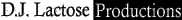Flight of the BumblebeeWho is on now? Guests: 203 Members: 1HomepageAccount

My Account
Inbox
Compose
New UserTabs Listing

List All Tabs
Tabs by Letter
View Files
Search Tabs
Random TabContribute

Forum
My Drafts
Post a FileResources

Site Blog
Tab Guide
Player List
Member List
Member Map
Tab Rulers
Mobile Site
Tab ToolWebsite

StoreAdd to Favorites | Post File | Print | Send to a Friend | Report Tab

 Tweet Song Name: Flight of the Bumblebee By: Rimsky/Korsakov Posted By: gene Difficulty: Expert Key: C Genre: General Harp Type: Chromatic Audio: Flight of the Bumblebee Created: 2009-02-06 02:02:03 Modified: 0000-00-00 00:00:00 Rating: Login to Vote Avg Rating: More Votes Needed Fav Count: 11
 Ad: Want to make Flight of the Bumblebee sound awesome on your harmonica? Master tight single notes, rock solid bending and more with easy video lessons ->click here.

 Song: 16 hole "C" Chromatic            14  -13<  -13  12<  13  12<  12  -12  12  -12  -11<  -11 11<  11  -10<  -10  10  -9<  -9  8<  -9  8<  8  8  -8  8  -8 -7 < -7 7<  7  -6<  6  6  -5<  -5  4<  -5  4<  4  -4  6  -5<  -5  4< -5  4<  4  -4  6  -5<  -5  4<  4  -6  6  -5<  6  -5<  -5  4< 4  4<  -5  -5<  6  -5<  -5  4<  4  -6  6  -5<  6  -5<  -5  4< 4  4<  -5<  5  6  -5<  -5  4<  -5  4<  4  -4  4  4<  -5  -5< 6  -6  6  -5<  6  -5<  -5  4<  -5  4<  4  -4  4  4<  -5  -5< 6  -6<  7  7<  -7  7<  7  -6<  -6  -7<  7  -7  7<  -7  7<  7  -6< -6  -6<  7  7<  -7  7<  7  -6<  -6  -7<  -7  7<  -7  7<  7  -6< -6  -6<  7  7<  -7  7<  7  -6<  7  -6<  -6  6  -6  -6<  7  7< -7  -7<  -7  7<  -7  7<  7  -6<  7  -6<  -6  6  -6  -6<  7  7< -7  -7<  -7  -7  -11  -7  -7  -11  -7  -7  -7<  -11<  - 7< -7<  -11<  -7<  -7<  -7  -7  -11  -7  -11  -7  -11  -7  -7<  -11<  -7< -11< -7<  -11<  -7<  -11<  -7  -7<  -7  -7<  -7  7<  -7  -7<  -7  -7<  -7 7< -7  -7<  -7  7<  -7  -7<  -8  8  8<  8  -8  -7<  -7  -7<  -8  8 8<  8   -8  -7<  -7  -9  -5  -9  -5  -9  -5  -5<  -5<  -9<  -5< -9<  -5<  -9<  -5<  -5  -5  -9  -5  -9  -5  -9  -5  -9<  -9<  -5< -9< -5<  -9<  -5<  -9<  -9  -9<  -9  8<  -9  -9  -9  8<  -9  -9<  -9  8< -9  -9<  -9  8<  -9  -9<  10  -10  -10<  -10  10  -9<  -9  -9<  10 -10 -10<  -10  10  -9<  -9  8<  8  -8  -7<  -13<  -13  12<  -13  12<  12 -12 -11<  -12  12  12<  -13  12<  12  -12  12  -12  -11<  -11  -11<  -12 12  12< 12  12<  -13  -13<  14  -13<  -13  12<  -13  12<  12  -12  12  -12 -11<  -11 11<  11  -10<  -10  10  -10  10  -9<  10  -10  10  -9<  10  -10  10 -9< 10  -10  10  -9<  10  -10  10  -6  6  -5<  6  -6  6  -5< 6  -6  6  -5<  6  -5<  -5  4<  -5  4<  4  -4  4  -4  -3<  -3 3<  3  -2<  -2  2  -2  2  -1<  2  -2  2  -1<  2  -2  2  -1< 2   -2   2  -1<  2  -2  2  -1<  2  -2  2  -1<  2  -2  2  -1< 2   -2   2  -1<  2  -2  -2<  3  3<  -3  -3<  -4  4  4<  -5  -5< 6  -6  -6<  7  7<  -7  -7<  -8  8  8<  -9  -<9  10  -10  10  -9< 10  -10  10  -9<  10  -9<  -9  8<  8  -10  10  -9<  10  -9<  -9  8< 8  8< - 9  -9<  10  -9<  -9  8<  8  -10  10  -9<  10  -9<  -9  8< 8  8<  -9  -9<  10  -9<  -9  8<  -9  8<  8  -8  8  8<  -9  -9< 10  -10  10  -9<  10  -9<  -9  8<  -9  8<  8  -8  8  8<  -9  -9< 10  -10<  11  11<  -11<  11<  11  -10  -10<  -11<  -11  11<  -11  11< 11  -10< -10  -10<  11  11<  -11  11<  11  -10<  -10  -11<  -11  11<  -11  11< 11  -10< -10  -10<  11  11<  -11  11<  11  -10<  11  -10<  -10  10  -10  -10< 11  11< -11  -11<  -11  11<  -11  11<  11  -10<  -10  -10<  11  11<  -11  -12 12  -13 14  -14  14  -13<  14  -13<  -13  12<  12<  -14  14  -13<  14  -13< -13  12< 12<  -13  -13  -13<  14  -13<  -13  12<  12<  -14  14  -13<  14  -13< -13  12< 12  12<  -13  -13<  14  11<  -11  -11<  -12  12  12<  -13  12<  12 -12 12  -12  -11<  -11  11<  -11  -11<  -12  12  12<  -13  -13<  14  -14 14  -13< 14  -14  14  -13<  14  11<  -11  -11<  -12  12  12<  -13  12<  12 -12 12  -12  -11<  -11  11<  -11  -11<  -12  12  12<  -13  -13<  14  -14 14  -13< 14  -14<  15  15<  -15  15<  15  -14<  15  -14<  -14  14  -14  14 -13<  -13 12<  12  -12  -11<  -11  11<  11  -10<  11  -10<  -10  10  -10  10 -9<  -9 8<  8  -8  -7<  -7  -7<  -7  7<  -7  -7<  -7  7<  -7  -7<  -7  7< -7  -8  8<  -9  10  -10  10  -9<  10  -10  10  -9<  10  -10  10  -9< 10  -10<  11  11<  -11  6  -6  -6<  7  7<  -7  -7<  -8 8  8<  -9  -9<  10  -10  -10<  11  11<  -11  -11<  -12  12  12<  -13 -13< 14  -14<  15  15<  -15  -7 - 3

Users who have Favorited this tab
 Username 1 03lindsi 2 aussie 3 aztim 4 docsoc11 5 EarthDogHarpin 6 HarvsHarp 7 jolimzw 8 KPHCreationz 9 lucask 10 Macaubeiro 11 underdog